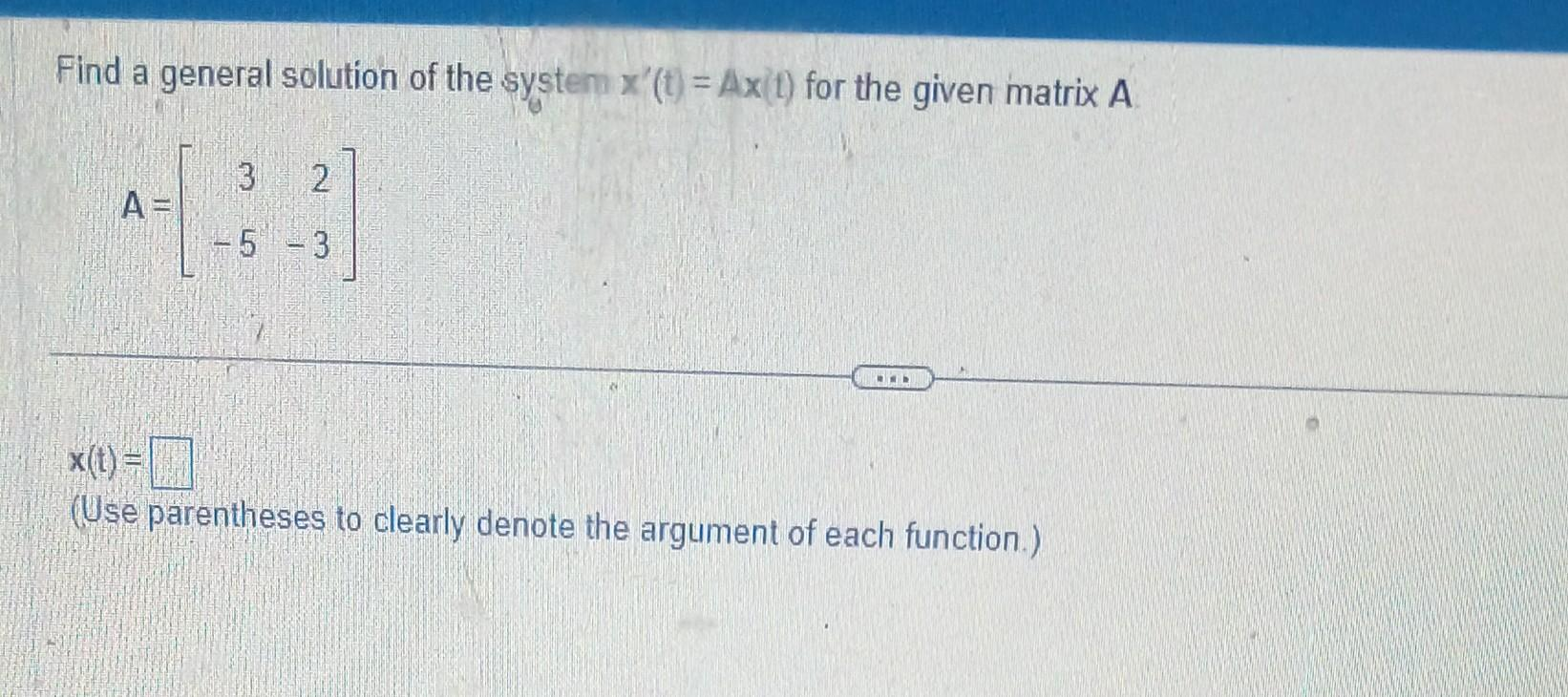# (Solved): Find a general solution of the systen x(t)=Ax(t) for the given matrix A A= ...Find a general solution of the systen for the given matrix (Use parentheses to clearly denote the argument of each function.)

We have an Answer from Expert